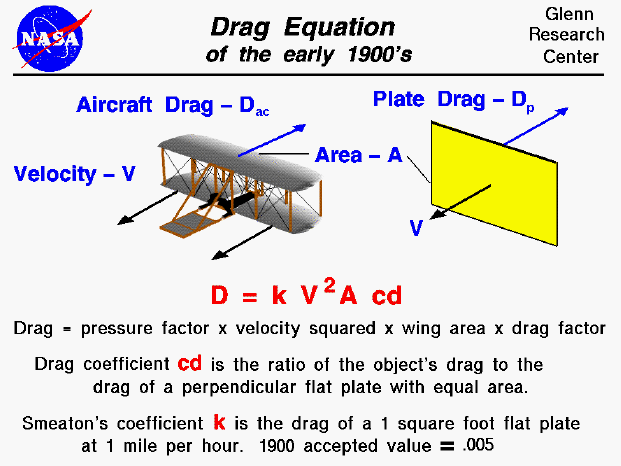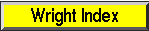+ Text Only Site
+ Non-Flash Version
+ Contact GlennBetween 1900 and 1905, the Wright brothers designed and built three unpowered gliders and three powered aircraft. In the design of each aircraft, the brothers tried to maximize the lift to drag ratio because high lift and low drag gives the best airplane performance. How did they predict the lift and drag of their design? The Wright brothers were bicycle mechanics and designers and they had a good working knowledge of math and science. They knew about Newton's laws of motion and about forces and torques. They had written to the Smithsonian when they began their enterprise in 1899 and received technical papers describing the aeronautical theories of the day. There were mathematical equations which could be used to predict the amount of lift and drag that an object would generate. The drag equation is shown on this slide. The amount of drag generated by an object depends on a number of factors, including properties of the air, the velocity between the object and the air, the surface area over which the air flows, the shape of the body, and the body's inclination to the flow, also called the angle of attack. By the time the Wrights began their studies, it had been determined that drag depends on the square of the velocity and varies linearly with the surface area of the object. Early aerodynamicists characterized the dependence on the properties of the air by a pressure coefficient called Smeaton's coefficient which represented the pressure force (drag) on a one foot square flat plate moving at one mile per hour through the air. They believed that any object moving through the air converted some portion of the pressure force into drag, and they represented that portion by a drag coefficient. The resulting equation is given as: D = k * V^2 * A * cd where D is the drag, k is the Smeaton coefficient, V is the velocity, A is the wing area, and cd is the drag coefficient. This equation is slightly different from the modern drag equation used today. The modern equation uses the dynamic pressure of the moving air for the pressure dependence, while this equation uses the Smeaton coefficient. Modern drag coefficients relate the drag force on the object to the force generated by the dynamic pressure times the area, while the 1900's drag coefficients relate the drag force to the drag of a flat plate of equal area. For the modern drag equation the drag coefficient of a flat plate moving perpendicular to the flow is 1.28; for the 1900's drag equation, the drag coefficient for this problem is equal to 1.0. The 1900's equation assumes that you know the perpendicular pressure force on a moving flat plate (Smeaton coefficient). Because of measuring inaccuracies at the time, there were many quoted values for the coefficient ranging from .0027 to .005. Lilienthal had used the .005 value in the design and testing of his wings. When the Wrights began to design the 1900 aircraft, they used values for the drag coefficient based on the work by Lilienthal so they too used the .005 value. During the kite and glider experiments of 1900 and 1901, the brothers measured the performance of their aircraft. Neither aircraft performed as well as predicted by the lift and drag equations. The 1901 aircraft had been designed to lift itself (100 pounds) plus a pilot (150 pounds) when flown as a kite in a 15 mile per hour wind at 5 degrees angle of attack. But in flight, it could barely lift itself in a 15 mile per hour wind at a much higher angle of attack. So the brothers began to doubt the .005 value for the Smeaton coefficient and they determined that a value of .0033 more closely approximated their data. The modern accepted value is .00326. The brothers also began to doubt the accuracy of Lilienthal's lift and drag coefficients. So in the fall of 1901, they decided to determine their own values for the drag coefficient using a wind tunnel. The brothers built a clever balance to directly measure the ratio of the drag of their models to the lift of the model. They built another balance to determine the lift. We have developed an interactive tunnel simulator so that you can duplicate their wind tunnel results. In the process of testing many airfoil models, the brothers discovered the importance of wing shape on the drag coefficient. They determined that the Lilienthal data was correct for the wing geometry that he had used, but that the data could not be applied to a wing with a very different geometry. Lilienthal's wings had a rather short span and an elliptical planform, while the brothers used a long, thin, rectangular planform. The brothers tested over fifty different models to determine how lift and drag are affected by various design parameters and they used this data to design their 1902 aircraft using the drag equation shown on the slide with their own drag coefficients. The brothers determined the power requirements for the 1903 aircraft based on their drag data. You can view a short movie of "Orville and Wilbur Wright" discussing the drag force and how it affected the flight of their aircraft. The movie file can be saved to your computer and viewed as a Podcast on your podcast player. Navigation..Beginner's Guide Home Page+ Inspector General Hotline + Equal Employment Opportunity Data Posted Pursuant to the No Fear Act + Budgets, Strategic Plans and Accountability Reports + Freedom of Information Act + The President's Management Agenda + NASA Privacy Statement, Disclaimer, and Accessibility CertificationEditor: Nancy Hall NASA Official: Nancy Hall Last Updated: May 13 2021 + Contact Glenn